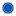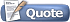Thread: [Math] Summation Help View Single Post04-24-2014, 04:58 AM #5 emerald000 the Mathemagician~Join Date: Nov 2005 Location: Quebec City Age: 29 Posts: 1,320Re: [Math] Summation Help The formula only works for k=1. If you have a summation that starts from another number, you can split the summation: Sum(k to n) = Sum(1 to n) - Sum(1 to k-1)